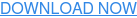### nuclear### chemical### Combustible dust### testing### news#### Recent Posts

By Elizabeth Raines, Chemical Engineer

....and Further Understanding the Differences between Reaction and Adiabatic Calorimetry

This article expands on the information presented inReaction Calorimetry vs. Adiabatic Calorimetry: Which Method is Right for Me?” and “Scale Up Aspects of Phenol-Formaldehyde Reactionsavailable on the Fauske & Associates, LLC website.

Thermal hazards screening is a quick and cost-effective tool used to obtain the required data for the safe scale-up of new or altered chemical processes. One parameter often used to understand the specifications of equipment required for large-scale manufacturing is the heat of reaction. While general studies found in literature can be useful for screening thermal hazards, oftentimes the characteristics of a particular reaction and reaction conditions are not available, and must be determined experimentally. Fauske & Associates, LLC (FAI) employs many tools to collect this necessary data including both reaction and adiabatic calorimeters. It was previously discussed in “Reaction Calorimetryvs. Adiabatic Calorimetry: Which Method is Right for Me?” the primary differences between the two types of instruments. The objective of this discussion is to give an example of one such difference with special emphasis on how to use adiabatic calorimetry to calculate the heat of reaction. The heat of reaction measured here is indicative of the process heat that needs to be handled in the plant as opposed to solely the theoretical heat of reaction since factors such as addition temperature can alter results.

As discussed in “Scale Up Aspects of Phenol-Formaldehyde Reactions,” producing phenolic resins is a very old but still very active process in the industry. The chemistry has a wide variety of uses such as ablation, abrasives, coatings, composites, wood bonding, and much more. This example explores a generic semi-batch phenol formaldehyde process where a controlled addition of 37% aqueous formaldehyde was added to a mixture of phenol and 50% caustic (catalyst). The same recipe was tested in both the FAI VSP2TM and Mettler Toledo RC1. The VSP2 test was performed with a 50°C starting temperature while the RC1 was performed isothermally at 70°C.

Figures 1 and 2 show the temperature and temperature rise rate profiles from the adiabatic calorimeter experiment.Figure 1: Temperature vs. Time for the VSP2 Phenol Formaldehyde ReactionFigure 2: Temperature Rise Rate vs. Temperature for the VSP2 Phenol Formaldehyde Reaction

This data can be used to determine many important parameters such as the required relief area (vent sizing), characteristic time of adiabatic runaway, the moles of noncondensable gas generated, kinetic parameters, temperature of no return, self-accelerating decomposition temperature, heat of reaction, and others.

In order to calculate the heat of reaction, it is assumed that the heat of reaction and heat capacity are constant throughout the reaction, and that there is zero conversion of the limiting reagent at the onset temperature of reaction. Together with these assumptions, the following equation can be utilized to determine the heat of reaction.ΔΗr = the heat of reaction (J/g)

Φ = the phi-factor or thermal inertia for the experiment (-)

Cs = sample heat capacity (3.2 J/g-K)

ATR = the adiabatic temperature rise (K)

m = the mass fraction of limiting reagent (0,3 based on the mass of phenol)
mt

The phi-factor is specific to the experimental design. To calculate the phi-factor, the following equation can be utilized.Cs = sample heat capacity (3.2 J/g-K)

Cb= sample holder heat capacity (0.5 J/g-K)

ms = sample mass (74 g)

mb= sample holder mass (50 g)

For this experiment, the phi-factor is calculated to be 1.11

When external heating is not applied during the reaction, the adiabatic temperature rise, ATR, is the difference between the maximum temperature and the onset temperature. In this experiment, the reaction begins at 50°C when the addition is initiated. The maximum temperature observed in the experiment was 186°C, but the addition continued after it was realized. A cooling effect was observed bringing the temperature to 165°C at the end of the addition. Because this was the final temperature at the end of the reaction at known conditions (mass and heat capacity), 165°C is to be used as the maximum temperature. The ATR or difference between the maximum temperature and the onset temperature (165- 50°C) is 115°C. The phi-corrected ATR, (“ФATR)” , is found to be 128°C.

The results of this calculation indicate that for the measured ATR (128°C when phi-corrected) the heat of reaction for the phenol-formaldehyde reaction (both the initial and secondary reaction) is calculated to be 1,362 kJ/kg phenol.

The same reaction was studied in the RC1 (not shown in this article) and the measured heat of reaction was determined to be 982 kJ/kg phenol. The calculated ATR for the reaction was 95°C. Because the RC1 experiment was conducted at 70°C as opposed to the 50°C starting temperature in the VSP2, the sensible heat correctioin for the formaldehyde was adjusted in the RC1 to be representative of the VSP2 experimental conditions.

Why the difference? The RC1 was capable of controlling the reaction allowing only the desired reaction to occur. The VSP2 showed the total thermal potential when the reaction cannot be controlled (a loss of cooling scenario) resulting in more heat and a higher temperature rise. It is invaluable to have both pieces of this information to best prepare for how the desired process needs to be run and what could happen when an upset scenario occurs.